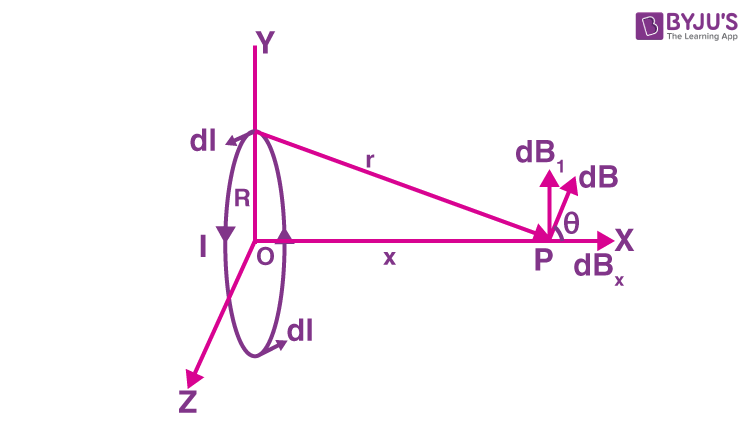# Magnetic Field on the Axis of a Circular Current Loop

We know that there exists a relationship between electricity and magnetism. The field around the magnet generates a magnetic field and the rotating magnets in a generator produce electricity. The phenomena which relates electricity and magnetism is known as electromagnetic force. In this article, let us learn about the magnetic field on the axis of a circular current loop in detail.

## Magnetic Field on the Axis of a Circular Current Loop – Derivation

Before we know more about the magnetic field on the axis of a circular current loop, let us understand the basic law of magnetism. We know that there exists an equation that describes the magnetic field generated by constant current. The Biot–Savart law correlates the magnitude of the magnetic field with the length, proximity, and direction of the electric current. The Biot–Savart law is an equation that gives the magnetic field produced by a current-carrying segment. The current-carrying element is considered a vector quantity. Biot–Savart law is given by the equation:

$$\left | dB \right |=(\frac{\mu _{0}}{4\pi })(\frac{Idlsin\Theta}{r^{2}})$$———–(1)

Where

μ0 is the permeability of free space and is equal to 4π × 10-7 TmA-1.

Now let us learn the derivation of a magnetic field on the axis of a circular current loop. Let us evaluate the magnetic field due to a circular coil along its axis. The evaluation requires summing up the effect of minute current elements (I dl). (I) is the steady current and the evaluation is carried out in a vacuum or free space.

Refer to the image given below. From the figure, we can see that (I) represents a circular loop carrying a steady current. From the figure, we can observe that the circular loop is placed in the y-z plane with its center at the origin O and has a radius R. The x-axis is the axis of the loop.

x = distance P from the center O of the loop.

dl= a conducting element of the loop.

The Biot-Savart law gives the magnitude of the magnetic field (dB) due to current element (dl)

$$\left | dB \right |=(\frac{\mu _{0}}{4\pi })(\frac{I\left | dl*r \right |}{r^{3}})$$

Now

$$r^{2}=x^{2}+R^{2}$$ ———–(2)

Any element of the loop will be perpendicular to the displacement vector from the element to the axial point.

For example, the element dl present in the figure is in the y-z plane. Whereas, the displacement vector r from dl to the axial point P is in the x-y plane.

Hence,

$$\left | dl*r \right |= r.dl$$

Thus,

$$\left | dB \right |=(\frac{\mu _{0}}{4\pi})\frac{Idl}{(x^{2}+R^{2})}$$

The direction of dB is as shown in the figure and is perpendicular to the plane formed by dl and r. It has an x-component $$dB_{x}$$ and a component perpendicular to x-axis, $$dB_{\perp}$$.

A null result is obtained when the components perpendicular to the x-axis are summed over, and they cancel out.

The $$dB_{\perp}$$ component due to dl is cancelled by the contribution due to the diametrically opposite dl element. This is represented in the above figure.

Hence, only the x-component survives. The net contribution along the x-direction can be obtained by integrating $$dB_{x}$$ = dB cos θ over the loop.

$$cos\Theta =\frac{R}{(x^{2}+R^{2})^{\frac{1}{2}}}$$ ———–(3)

From equation (2) and (3)

$$dB_{x}=\frac{\mu _{0}Idl}{4\pi }\frac{R}{(x^{2}+R^{2})^{\frac{3}{2}}}$$

The summation of elements dl over the loop yields 2πR, the circumference of the loop. Thus, the magnetic field at P due to the entire circular loop is

$$B=B_{x}\hat{i}=\frac{\mu _{0}IR^{2}}{2(x^{2}+R^{2})^{\frac{3}{2}}}\hat{i}$$

When x=0, we may obtain the field at the center of the loop

$$B_{0}=\frac{\mu _{0}I}{2R}\hat{i}$$

Since the circular wire forms a closed loop, the magnetic field lines are produced as shown in the figure below.The direction of the magnetic field created is given by the right-hand thumb rule.

### Practice Problem

1. A circular coil of wire has 100 turns of radius 12 cm, and carries a current of 1 A in a clockwise direction when viewed from the right side. Find the magnitude and direction of the magnetic field:

i) at the center of the coil, and

Given: N = 100, r = 0.12m, i = 1 A

Magnetic field at the center of the circular coil is given by:

$$B=\frac{\mu _{0}NI}{2R}$$ $$=\frac{4\pi *10^{-7}*100*1}{2*0.12}$$ $$=5.23*10^{-4} T$$

To learn about magnetic fields due to circular loops, click on the video below.Stay tuned with BYJU’S for more such interesting articles. Also, register to “BYJU’S – The Learning App” for loads of interactive, engaging Physics-related videos.

## Frequently Asked Questions – FAQs

### State right-hand thumb rule.

The right-hand thumb rule states that “Curl the palm of your right hand around the circular wire with the fingers pointing in the direction of the current. The right-hand thumb gives the direction of the magnetic field”.

### The equation that gives the magnetic field produced by a current-carrying segment.

The equation is given by the Biot savart law.
$$\left | dB \right |=(\frac{\mu _{0}}{4\pi })(\frac{Idlsin\Theta}{r^{2}})$$

### What is electromagnetic force?

It is one of the fundamental forces that deals with the physical interaction between electrically charged particles. The electromagnetic force acts between charged particles and is the combination of all magnetic and electrical forces.

### Give the equation for the magnetic field on the axis of a circular current loop.

$$B_{0}=\frac{\mu _{0}I}{2R}\hat{i}$$

### Is the current carrying element considered a scalar or vector quantity?

The current-carrying element is considered a vector quantity.